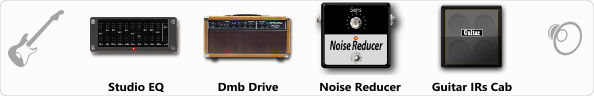# Dumble Brt. Crunch

Discussion in 'ToneLib-GFX presets' started by Jean C.Guion, Sep 26, 2019.

1. Dumble Brt. Crunch

Preset name: Dumble Brt. Crunch

Effects chain:Effect: "Studio EQ" (Dynamics / Filter), active - "yes"
"31 Hz" = 7
"62 Hz" = -4
"125 Hz" = 0
"250 Hz" = -5
"500 Hz" = -2
"1 kHz" = 0
"2 kHz" = -13
"4 kHz" = -7
"8 kHz" = 10
"16 kHz" = 12
"above 16 kHz" = 0
"Level (dB)" = -2

Effect: "Dmb Drive" (Amp simulators), active - "yes"
"Gain" = 88
"Bass" = 94
"Middle" = 47
"Treble" = 48
"Presence" = 38
"Master" = 60
"Output" = 50
"Level (dB)" = 11

Effect: "Noise Reducer" (Dynamics / Filter), active - "yes"
"Sens" = 14
"Mode" = Hard

Effect: "Guitar IRs Cab" (Cabinets), active - "yes"
"Model" = Marshall 1960A (4x12")
"Mic Position" = Middle
"Mic Distance" = Middle
"Low Cut (Hz)" = 51
"Hi Cut (kHz)" = 4.0
"Mix" = 100
"Level (dB)" = -1

Note: You will need to download and install the ToneLib-GFX software to use the preset.

File size:
447 bytes
Views:
3,392